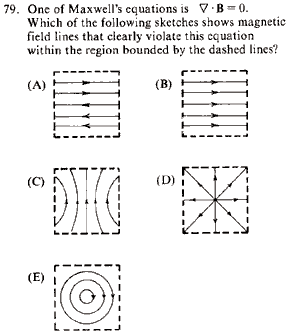GR 8677927796770177 | # Login | Register

GR8677 #79
Problem
 GREPhysics.NET Official Solution Alternate SolutionsElectromagnetism}Field Lines

The Maxwell Equation that states No monopoles" requires that the divergence of the magnetic field be 0, or more elegantly, . The problem asks for fields that violate this condition, so the condition to look for now is .

(A) ... divergence is 0

(B) ... divergence is 0

(C) this does not explicitly require the divergence to be 0.

(D) this is a non-zero divergence, as the field lines diverge outwards from a source.

(E) this is ... doesn't necessarily state anything aboutAlternate Solutions
 ramparts2009-11-06 10:39:09 Another way to think of this problem: grad B = 0 is the Maxwell's equations way of saying "no magnetic monopoles". D is the one that clearly shows a monopole being there :)Reply to this commentlivieratos
2011-11-08 06:10:01
i wonder how things would be affected if ever a magnetic monopole was discovered... would there just be a q_m (magnetic charge in a sense) on the right side of the equation or would things be much more complicated than that...ramparts
2009-11-06 10:39:09
Another way to think of this problem: grad B = 0 is the Maxwell's equations way of saying "no magnetic monopoles". D is the one that clearly shows a monopole being there :)
 IRFAN 2011-08-18 03:10:32 plz use div.B instead of grad b
 pam d2011-09-26 21:36:28 smh
 danielsw986672019-09-30 11:58:04 I definitely agree that D shows the monopole. Tampa SEO Companyanmuhich
2009-03-21 13:58:00
Have a visual understanding of what the "divergence" of a field is. D is the only answer which clearly has field lines which are diverging, wherease the other ones could curl back around on themselves eventually (or do).ArtifexR
2009-02-23 19:53:06
Another way to consider the problem is to remember that the equation is the equivalent of Gauss' Law for Magnetism. Hence, the total magnetic flux through any Gaussian surface must be 0. The only diagram which definitely has a flux through the walls of the box is diagram D.
 insertphyspun2011-02-21 19:52:31 Absolutely. The integral form of is derived using the divergence theorem. Thus, all lines within the boxes that are drawn must enter and leave. This is not true for D.ArtifexR
2009-02-23 19:52:34
Another way to consider the problem is to remember that the equation is the equivalent of Gauss' Law for Magnetism. Hence, the total magnetic flux through any Gaussian surface must be 0. The only diagram which definitely has a flux through the walls of the box is diagram D.T
2005-11-08 21:20:01LaTeX syntax supported through dollar sign wrappers $, ex.,$\alpha^2_0$produces . type this... to get...$\int_0^\infty\partial\Rightarrow\ddot{x},\dot{x}\sqrt{z}\langle my \rangle\left( abacadabra \right)_{me}\vec{E}\frac{a}{b}\$